# Finding the final y-position of a projectile

• Qube

#### Qube

Gold Member
Summary:: After firing a projectile from the top of a hill, where does it land on the hill? I disagree with the professor in approach and final result.

Problem Statement: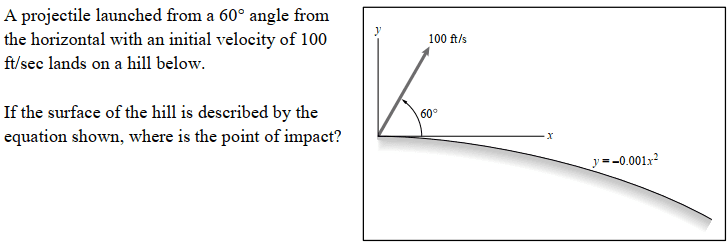Relevant Formulas: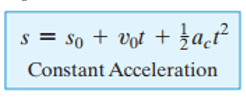Attempt to Solve:

I'm very familiar with these types of problems. I'm not here for help solving the entire problem. I just have a small point of contention with my professor about finding the final y-coordinate of the projectile.

I am confident that to find the final y-coordinate of the projectile, the correct equation should be: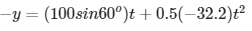My professor argues that the correct equation is instead: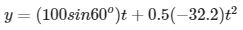We both agree on the equation for finding the x-coordinate of the projectile.

I disagree with the professor's equation for finding the final y-coordinate of the projectile. Displacement is final position - initial position, and the final y-coordinate is obviously "-y." The initial y-coordinate is obviously 0. The displacement of the particle, therefore, should be "-y" not "y."

Also, solving similar problems in the textbook with the professor's approach results in negative time values.*

Question:

Who's formula is correct in solving for vertical displacement?

----

*Example of me getting a negative time value with the professor's approach:

This is the book's solution; note the "-y."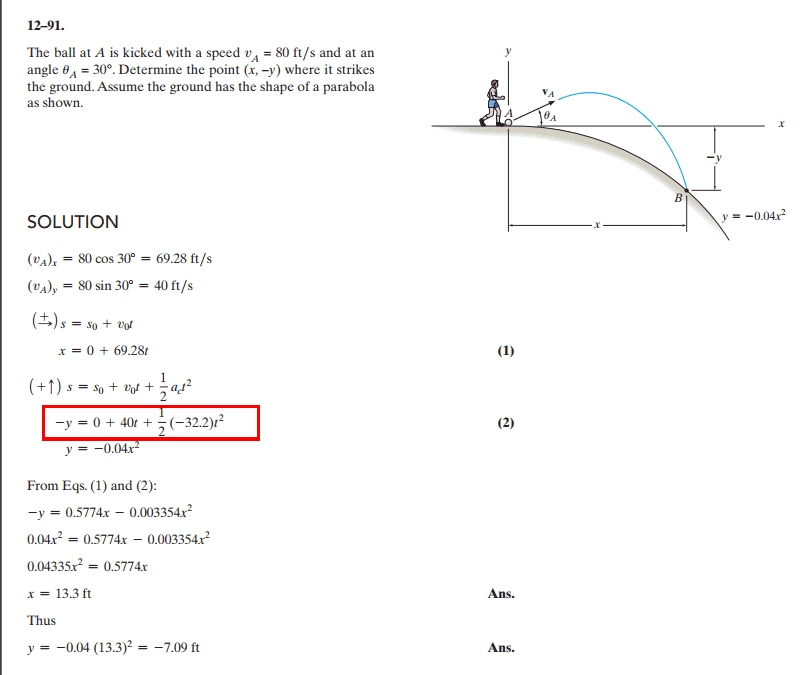This is what happens when you decide to solve it with the same equations, but with a "+y":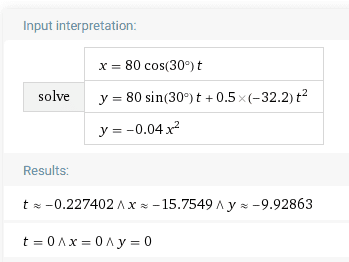Finally, this guy seems to have no issue with assuming the sign of the "y" in another similar problem: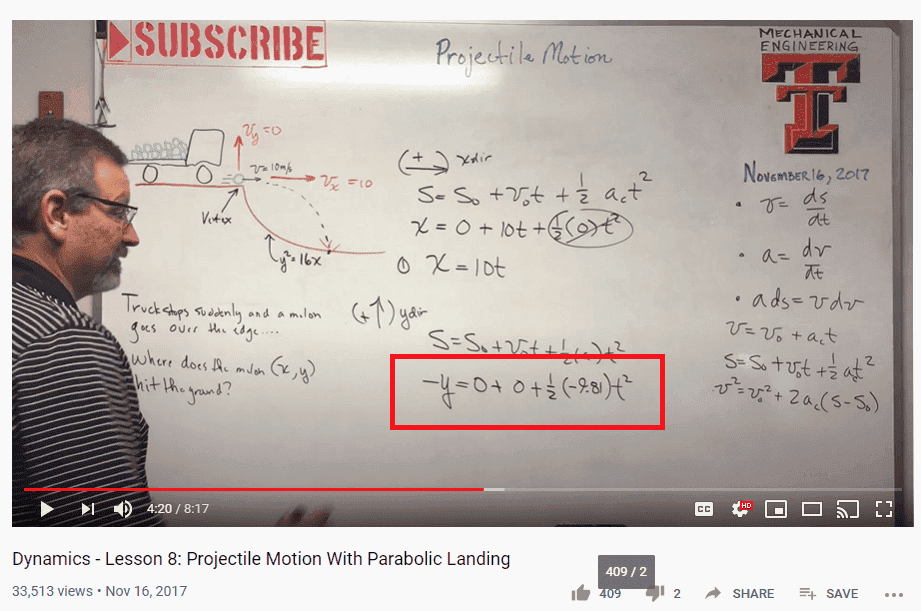Last edited:

Show me the points of impact of your way and of your teachers' way, if you please.

•Qube and berkeman
Here's a much more condensed version of what I'm asking: who's correct, the book or the teacher's method?

The points of impact are are in x and y. Time is "t."

I am not sure but I tend to agree with your teacher. I don't understand why we get a negative value for time though, the resulting equation for t will be a quadratic and most likely will have a positive root as well.

My main argument is that since your book says that the point of impact is (x,-y) then this point should satisfy the equation of the parabola so we should get the equation ##-y=-0.04x^2## and not ##y=-0.04x^2## as your book says. It is obvious to me that book is wrong here.

•A.T.
The displacement of the particle, therefore, should be "-y" not "y."
y(t) is, by definition, the displacement at time t.
If you take displacements as up positive then the displacement when it lands will have a negative value. You are confusing the sign of the variable in the equation with the sign of its value in the answer.

the final y-coordinate is obviously "-y."
This seems to be the core of the confusion. As @haruspex notes, the y-coordinate is simply "y". The term "-y" is the negated y-coordinate. If the book asks for "-y", then you can of course give them that, but don't call it "y-coordinate".

If the book asks for "-y", then you can of course give them that, but don't call it "y-coordinate".

Thanks, so what's the takeaway here for finding the final position of a particle that lands on a hill?

We have the book, which asks for "(x,-y)," and the book comes up with the "-y" displacement equation.

We have a famous professor on YouTube solving for "(x,y)" and he comes up with the same "-y" displacement equation.

And then we have my professor who wants me to solve it with the "+y" displacement equation.

Can someone help clarify what the takeaway should be?

We have a famous professor on YouTube solving for "(x,y)" and he comes up with the same "-y" displacement equation.
This fellow seems to have defined down as positive for the y displacement. I would guess this is because he also has y in a formula for a parabola which dips down from the x axis.
The formula "##-\frac 129.8t^2 ms^{-2}##" gives the displacement under gravity on the assumption that up is positive. Hence he needs to put in a minus sign somewhere. I would have written his equation as ##y=\frac 129.8t^2 ms^{-2}##, but he chose to put the extra minus on the left. Confusing.
Better still, I would have stuck with up as positive for y but written ##y=-\frac 129.8t^2 ms^{-2}## for the trajectory of the truck and ##y=-4\sqrt x## for the trough in the road.

The takeaway message is that you are free to define the positive direction for each of your variables as you wish, but you will confuse yourself less if you stick to common usage (up and right positive), and you should write down anything nonstandard.

•Qube, A.T. and Delta2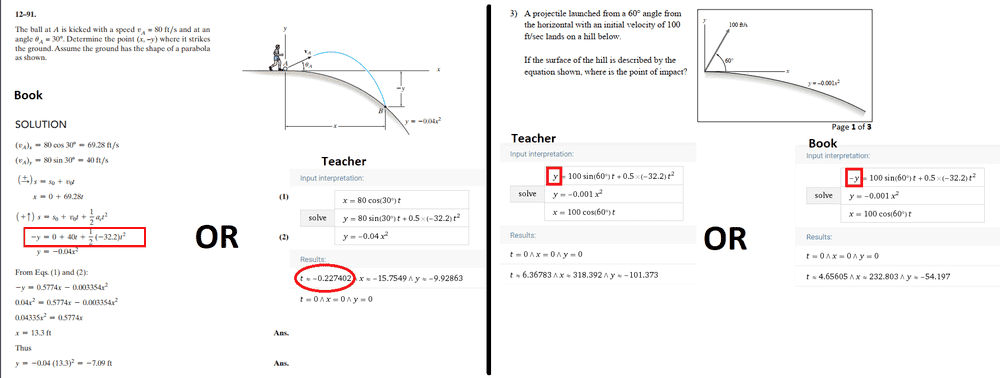It is obvious to me that book is wrong here.
I agree. The book computes the intersection of the ground surface with the vertically mirrored trajectory, not with the actual trajectory.

That's the only reason why they get a solution with positive time given their numbers (left half of the image above). The correct answer is that there is no impact, because the ground surface bends down stronger than the trajectory.

In the other problem (right half of image above) both approaches give a solution. The wrong book approach gives a shorter time, because the vertically mirrored trajectory intersects the ground surface earlier than the actual trajectory.

Last edited:
•Qube and Delta2